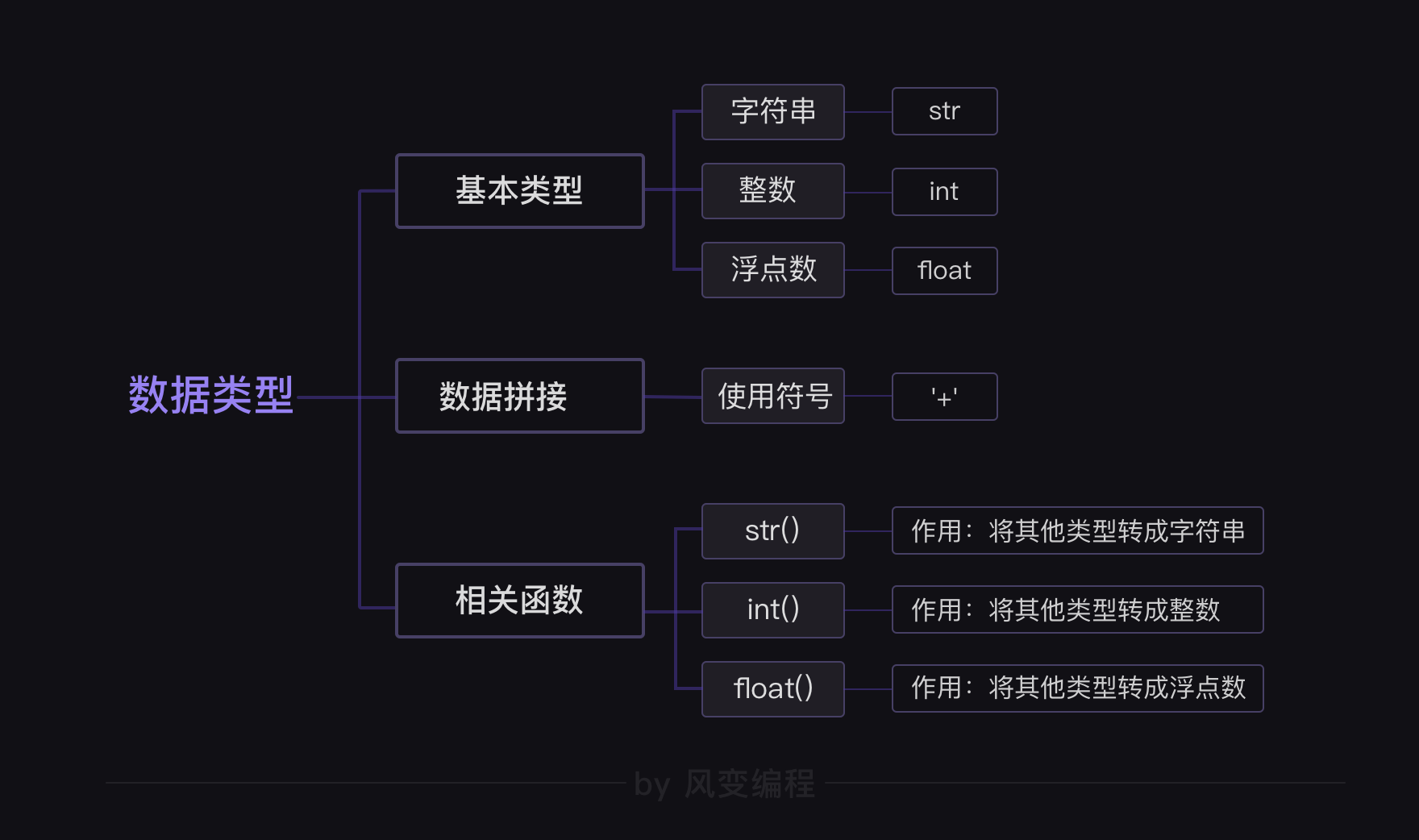## 一.数据类型

#### 1.字符串

``````print('来呀，打我呀，你们抓不到我的！')
print('保护我方鲁班七号！')
print('Double Kill!')
print('10s')

slogan = '来呀，打我呀，你们抓不到我的！'
alarm = '保护我方鲁班七号！'
achieve = 'Double Kill!'
cooling = '10s'

print(slogan)
print(alarm)
print(achieve)
print(cooling)
``````

#### 2.整数

2.1单独整数可以直接打印，不用带引号

``````print(666)
#打印整数

666
#打印结果
``````

2.2 整数可以直接进行运算

``````print(499*561+10620-365)
print((5025-525)/100+18*17)
``````

Python的运算符在写法上，与我们平时使用的运算符稍微有点区别。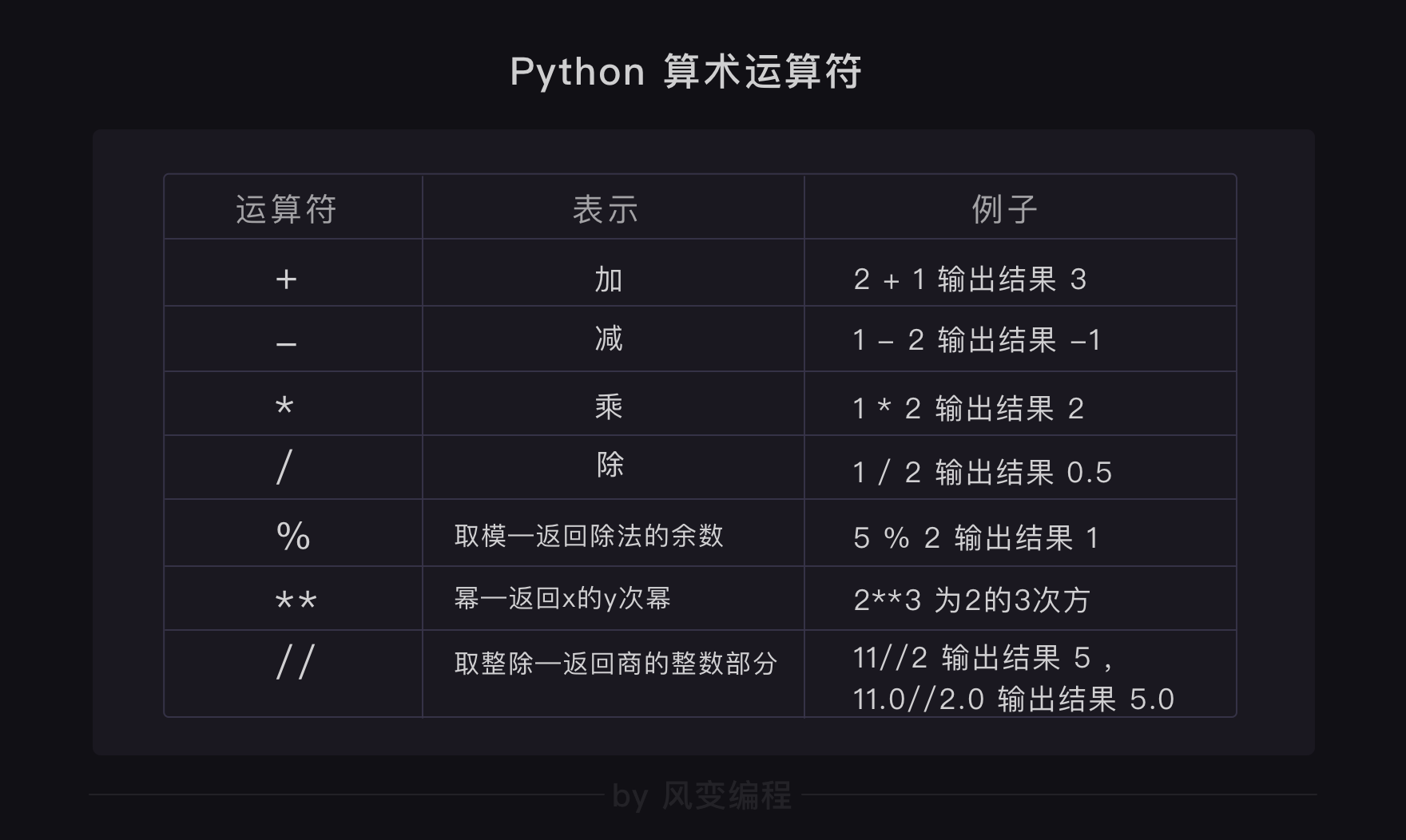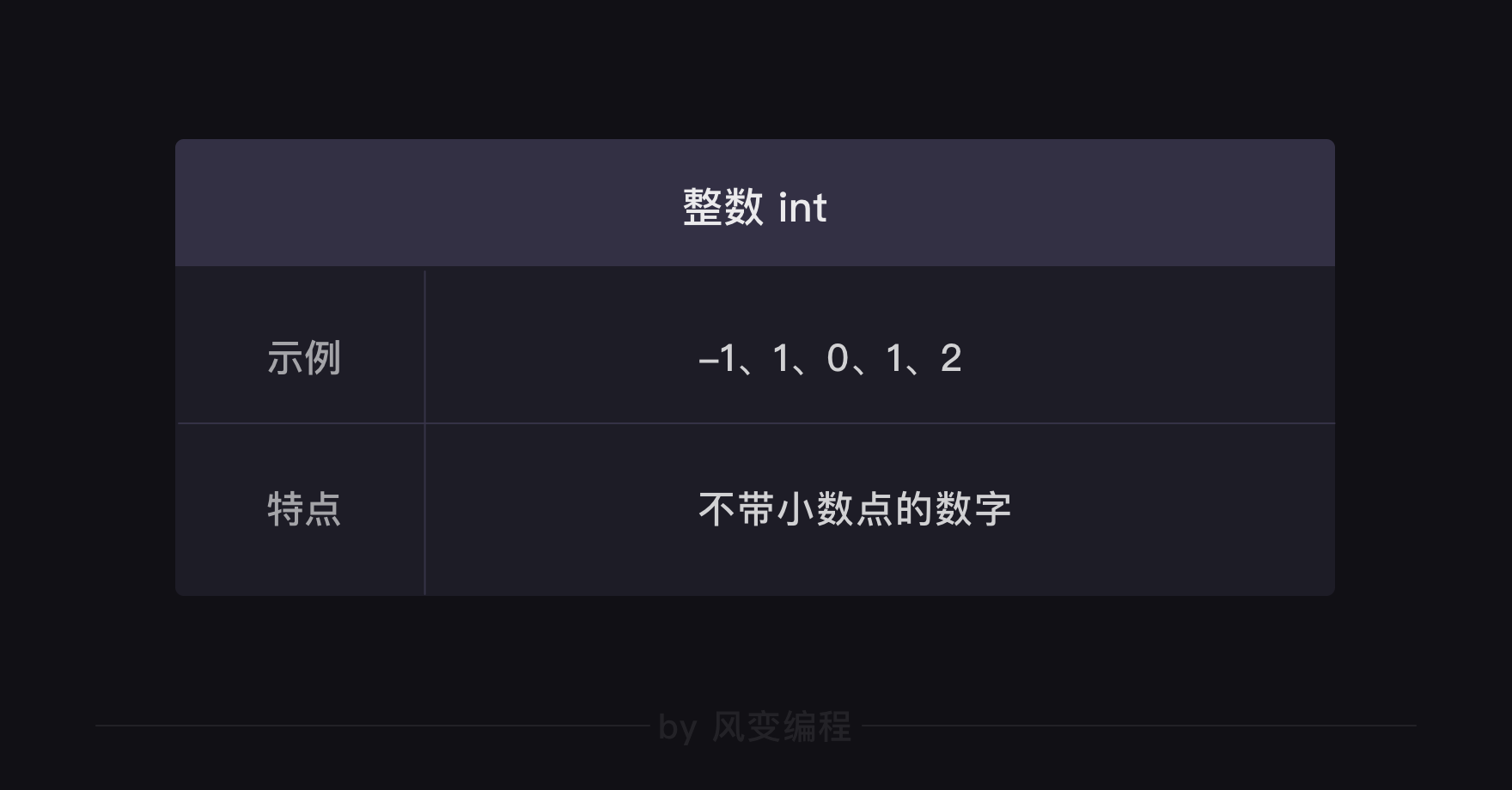### 3.浮点数

``````1.0
3.14159
-0.33
``````

#### 浮点数知识点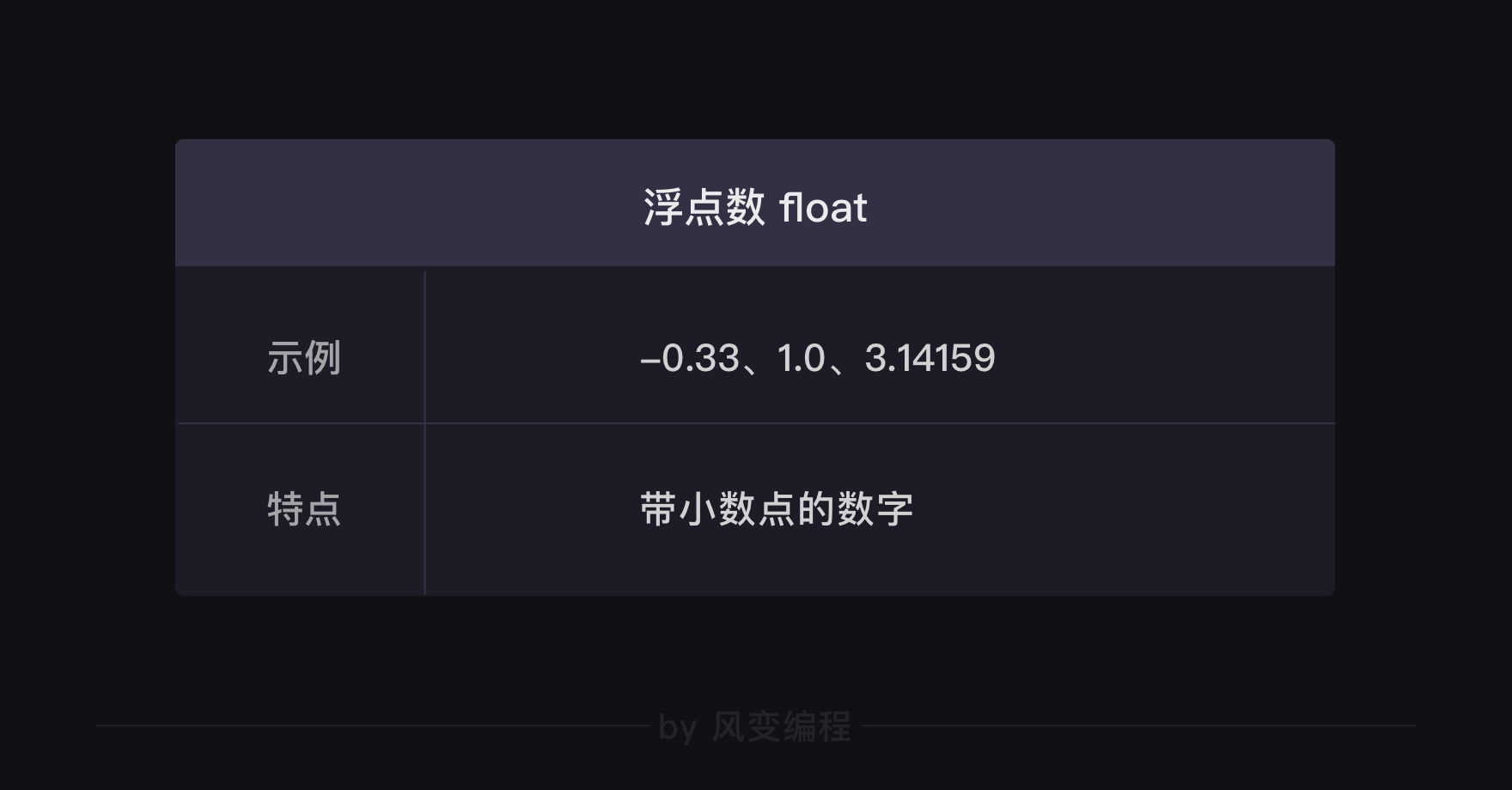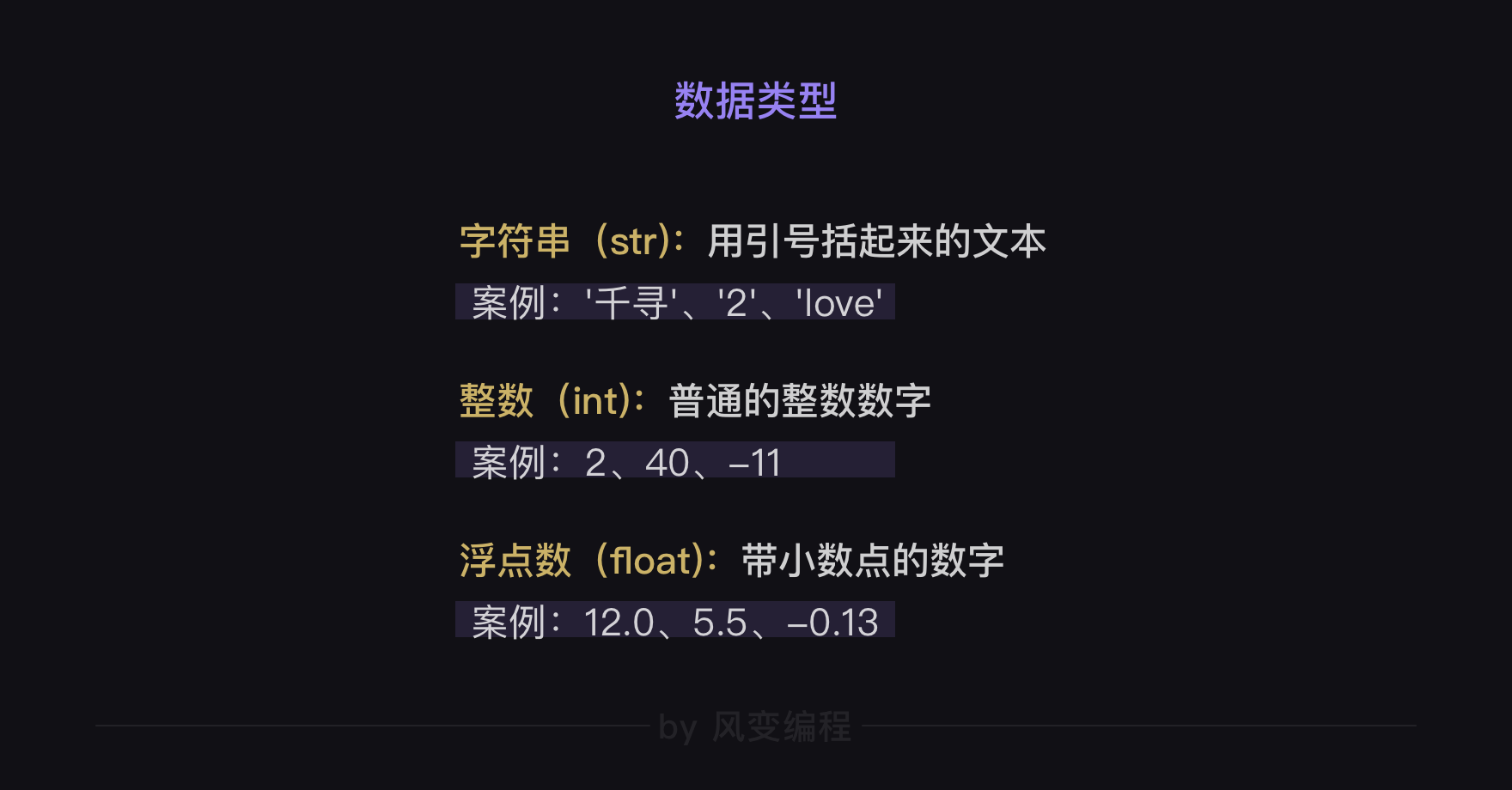## 二、数据拼接

``````hero = '亚瑟'
enemy = '敌方'
action = '团灭'
gain = '获得'
achieve = 'ACE称号'

print(hero+action+enemy+gain+achieve)
``````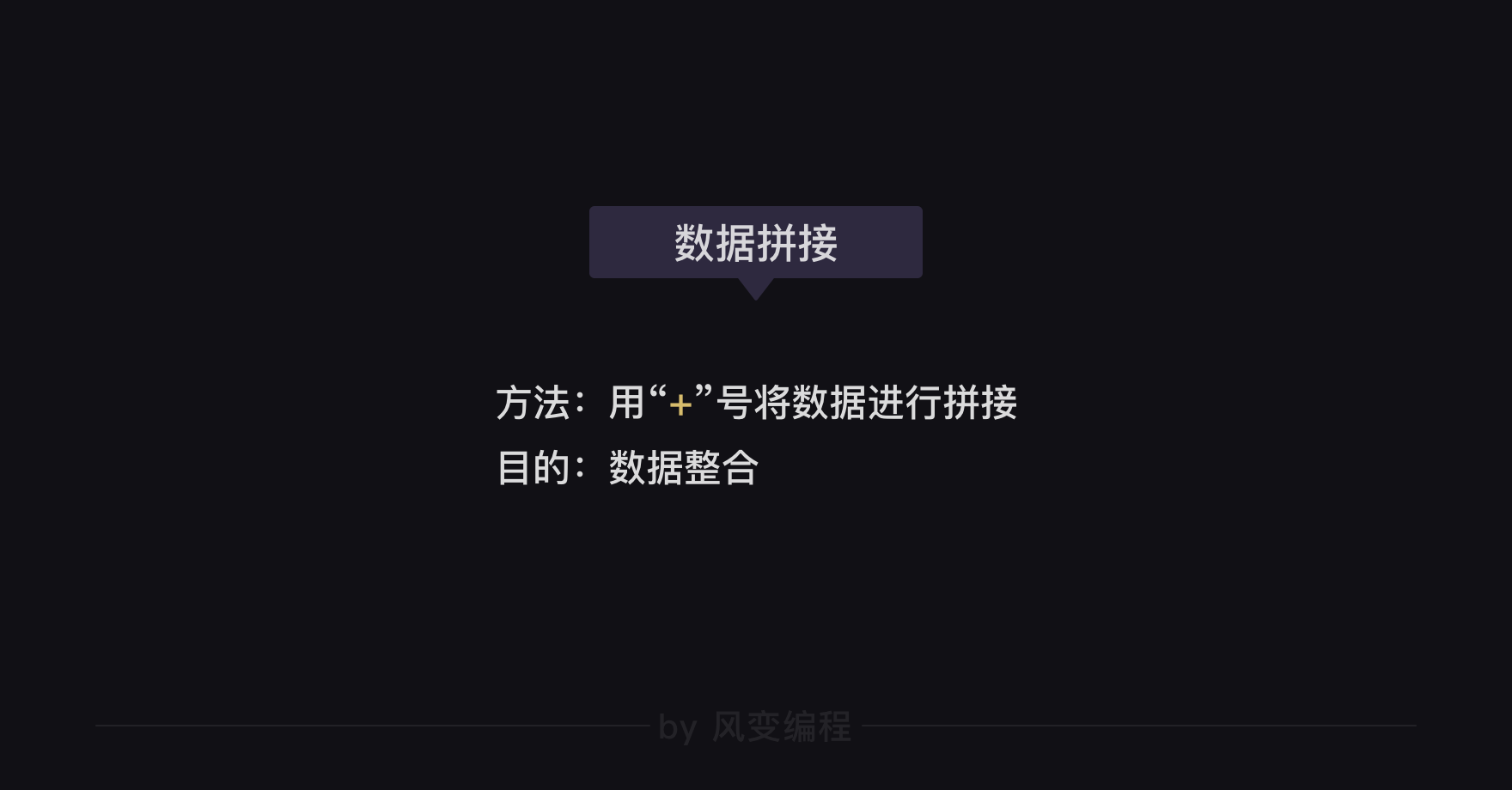### 2.1  type()函数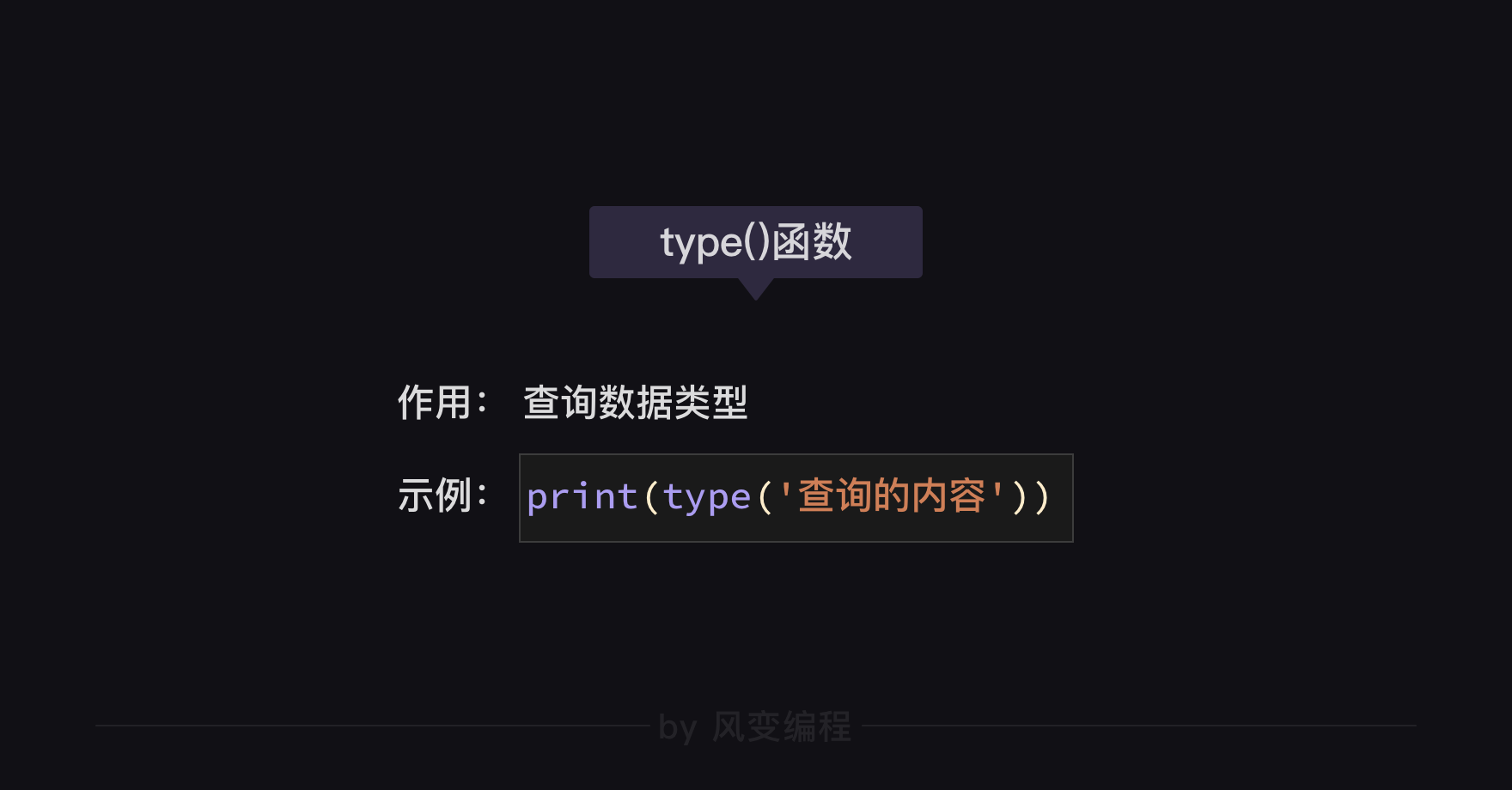## 三.数据转换

### 3.1  str()函数

``````hero = '亚瑟'
enemy = '敌方'
action = '秒杀'
gain = '获得'
number = 5
achieve = 'Penta Kill'

print(hero+action+str(number)+enemy+gain+achieve)
``````

``````slogan = '我一个电话立马有'
character = '山兔'
unit = '只'
place = '在你家楼下'
action = 'hola hola'
number = 10000

print(slogan+str(number)+unit+character+place+action)
print(slogan+'10000'+unit+character+place+action)
``````

### 小结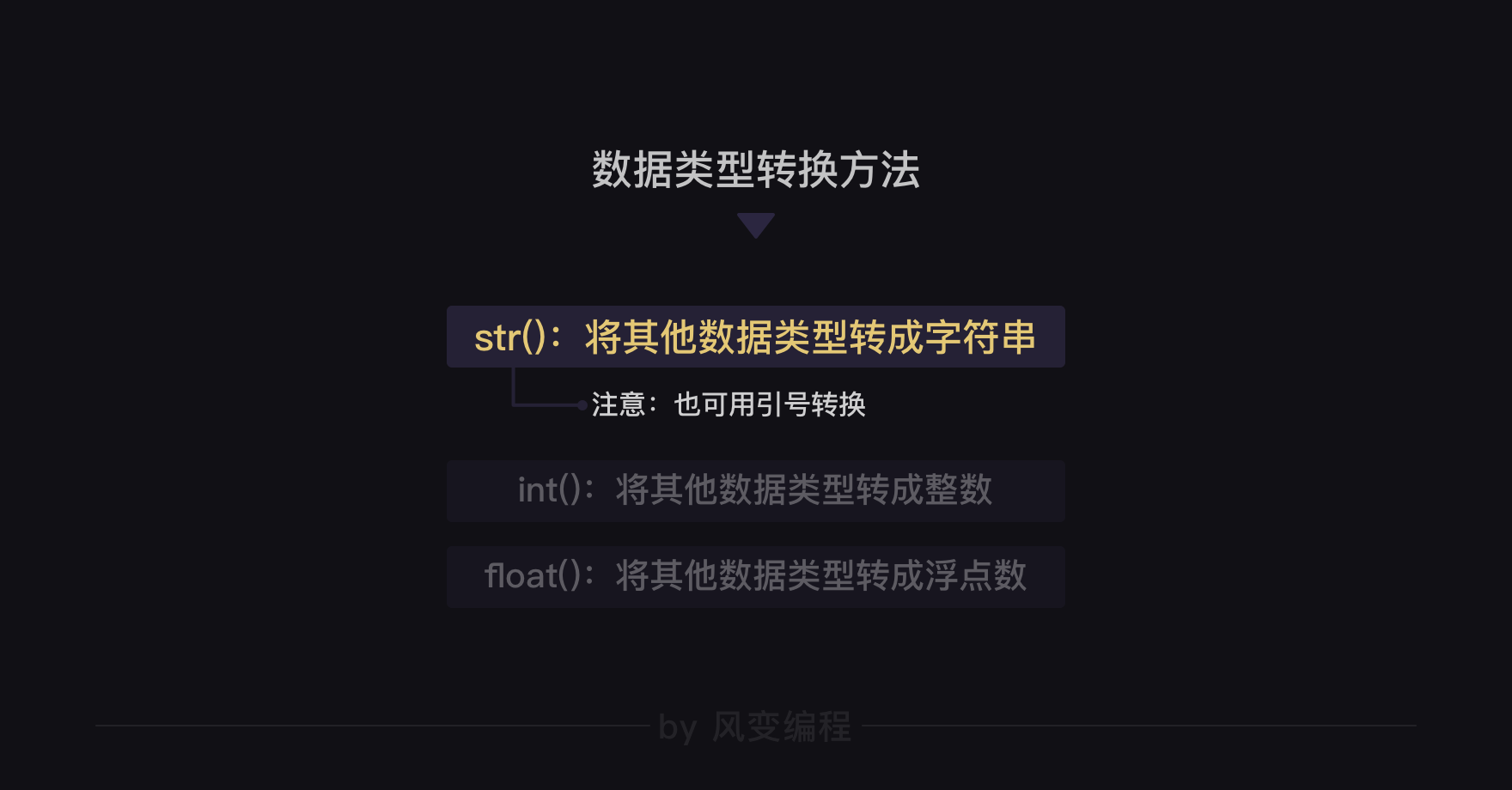### 3.2  int()函数

int()函数。其使用方法同str()一样，将你需要转换的内容放在括号里就行，像这样：int(转换的内容)。

``````bug = '666'
hair = '0'
print(int(bug)+int(hair))
``````

int()函数的本质是将数据转换为整数。所以对于浮点数，int()函数会做取整处理。但是，同我们平时对小数四舍五入的处理方法不同，int()函数会直接抹零，直接输出整数部分。

``````print(int(3.8))

3
``````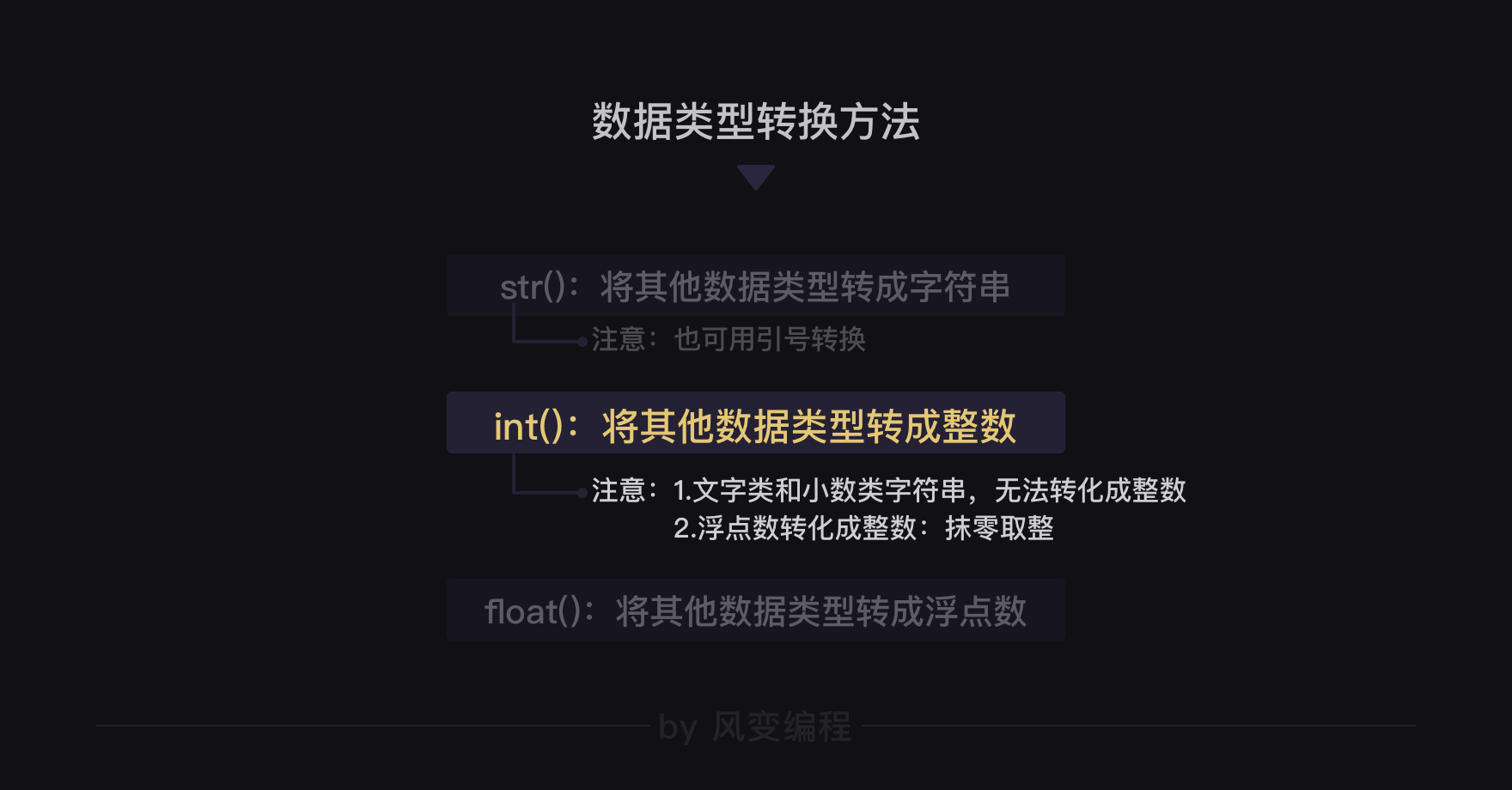### 3.3   float()函数

float()函数也可以将整数和字符串转换为浮点类型。但同时，如果括号里面的数据是字符串类型，那这个数据一定得是数字形式。

``````height = 183.5
weight = 79
age = '30'

print(float(height))
print(float(weight))
print(float(age))
``````

``````word = '3.8'
number = 1
sentence = '人工智障说：3.8+1等于'

print(sentence+str(int(float(word)+number)))